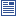Accessibility options:

# Introduction to Probability resources

Show me all resources applicable to

### 04. Tests and Quizzes (1)Business Statistics 2 - Numbas
5 questions. 1. Counting number of occurences of an event in a sample space with given size and finding the probability of the event. 2. Finding probabilities from a survey given a table of data. 3. Example showing how to calculate the probability of A or B using the law p(A or B)=p(A)+p(B)-p(A and B). Also converting percentages to probabilities. 4. Choose whether given three given pairs of events are independent or not. 5. Given data on probabilities of three levels of success of three options and projections of the profits that the options will accrue depending on the level of success, find the expected monetary value (EMV) for each option and choose the one with the greatest EMV. Numbas resources have been made available under a Creative Commons licence by the School of Mathematics & Statistics at Newcastle University.

### 07. Community Project (1)6 Probability (Workshop SOURCE)
This PowerPoint workshop provides a presentation on basic probability theory. This resource has been contributed to the statstutor Community Project by Peter Samuels, Birmingham City University under a Creative Commons licence CC-BY-SA and reviewed by Ellen Marshall, University of Sheffield. The zip file contains the source file and the associated statstutor metadata spreadsheet.

### 10. Workshops (1)6 Probability (Workshop)
This PowerPoint workshop provides a presentation on basic probability theory. This resource has been contributed to the statstutor Community Project by Peter Samuels, Birmingham City University and reviewed by Ellen Marshall, University of Sheffield.